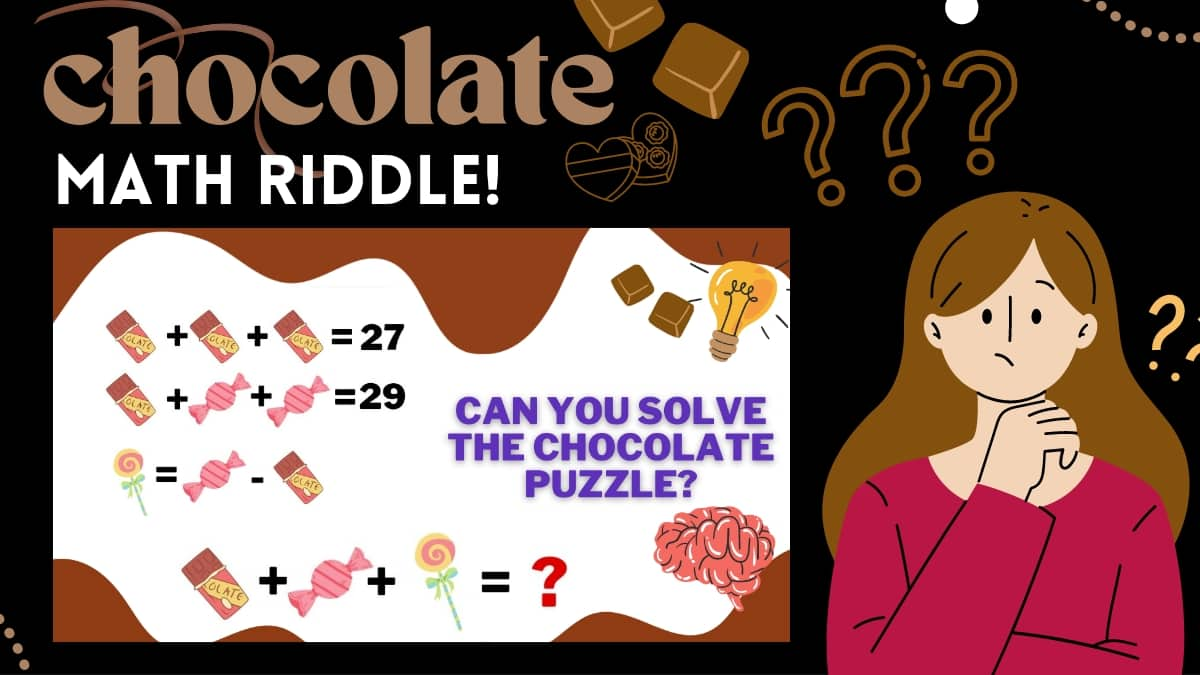# Math Riddles: Solve This Chocolate Puzzle In 30 Seconds And Test Your IQ!

Math Riddles Challenge: Can you solve this Chocolate Puzzle In 30 Seconds? Test Your IQ and sharpen your brain with this chocolatey maths riddle.Solve this Chocolate Puzzle In 30 Seconds And Test Your IQ!

Are Math Riddles your favorite just like chocolates? Then you will love this chocolate math puzzle! You need to find the value of each of these sweets and then their sum. Sounds like a challenge right? Can you do it in 30 seconds? Brush up your math skills, and analytical abilities and solve this savoring little puzzle filled with chocolates, candies, and lollipops!

## Here's your chocolate day puzzle!

Let’s Start.

Remember you have only 30 seconds!

Need a hint?

Look at the chocolates, they are all equal!

Could you solve it?

### Time's Up!

Could you get the correct answer?

## Let's solve it together

The first equation has three chocolate bars (CB) and their sum is 27.

By the logic Left Hand Side is equal to the right-hand side, we can find the value of the chocolate bar.

## First Equation:

### And 3 times 9 is 27, hence proved.

In the second Equation, we have 1 chocolate bar (CB) and two candies(C). Their sum is 29. Now that we know CB is equal to 9,  by the logic Left Hand Side is equal to the right-hand side, we can find the value of the candies as well.

## Second Equation:

### 9 added to 2 times 10 is 29, hence proved

Now that we have the value for both the chocolate bar and candy, the third equation is fairly easy where the lollipop(L) is equal to the value of candy (C) subtracted by the value of the chocolate bar(CB). Now that we know that C is equal to 10 and CB is equal to 9 we can find the value of the lollipop by the logic of LHS=RHS.

## L=1

Now comes the final equation, we know the value of the chocolate bar(CB), candy(C), and lollipop (L) we can easily find out their sum!

### The correct answer is 20.

Great work!

Fun right? I bet you want to reward yourself with a bar of chocolate now! Solve these puzzles everyday and sharpen your analytic skills without any fail.

Also Check out!

Math Riddles: Can You Solve This Heart Puzzle In 30 Seconds?

Math Riddles: Do You Have A High IQ? Solve These Figures and Find the Missing Number!

Math riddles with answers: Try these math riddles for a quick break!

Get the latest General Knowledge and Current Affairs from all over India and world for all competitive exams.
खेलें हर किस्म के रोमांच से भरपूर गेम्स सिर्फ़ जागरण प्ले पर